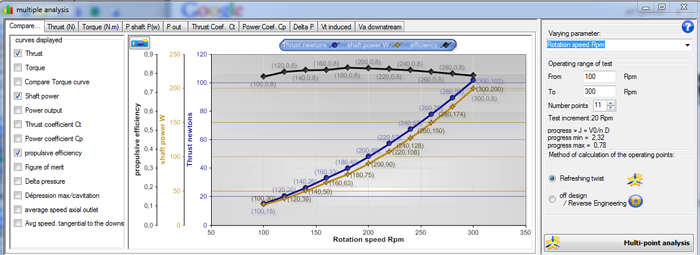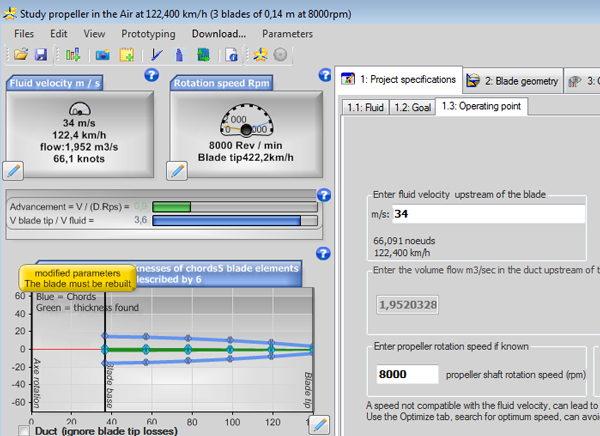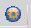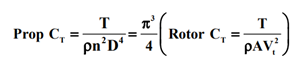Design: propeller turbine, screw, aerial, marine, turbine, tidal, wind, kaplan, foil, wings, 3D. Discover heliciel software: Modeling aerial screw propeller in heliciel Modeling a wing mast sail in heliciel Wings or foils modeling in heliciel
Propeller propulsion performance at zero speedWe have seen the different tutorials that we could build a propeller propulsion optimized for a given operating point (rotational speed and fluid speed) . (see Tutorial propeller aircraft design (1) et Tutorial boat propeller design (1) )
It is interesting to evaluate the performance of our traction propeller when the velocity is zero, which is the case when starting a boat or plane, or the hélicoptere in hovering flight.
• To test the performance at zero speed of a propeller designed for a given cruising speed,
1. we must have previously set the desired operating point for our propeller with cruising speed, and updated geometry by clicking rebuild. (cliick) (This has the effect of updating the twisting of our propeller blade optimized for the operating point described by the rotational speed and the fluid velocity2. We just then entering a speed of movement, almost zero (0.01 msec: zero fluid velocity is forbidden in the calculations, it is necessary to enter a value close to zero to simulate a zero speed) .
For the performance of the propeller without rebuilding twisting, use the calculation "off design ",This means that the performance will be calculated without rebuilding twisting. "Off design" mode is symbolized by a "HD"front of different results to recall that the twisting of the propeller is not optimal for the current operating point. The thrust and torque will be updated. You can vary your rotational speed and restart performance calculations "Off design" until obtaining the desired thrust.
• For a propeller that work only in zero speed (or almost zero) as a stationary hélicoptere for example, we will update the twist at the operating pointto almost zero speed.

Assess the performance at zero speed:

the notion of propulsive efficiency becomes unusable because the propulsive efficiency is calculated by multiplying the thrust by the fluid velocity. A zero speed propulsion efficiency is zero.

To appreciate and evaluate the quality of our propeller at zero speed the figure of merit will be used. The figure of merit used to characterize a propeller,stationary, upstream speed = 0 .

The source NASA / CR — 2017–219486, details some possible confusions between propellers and rotors in terms of nomenclatures, And warns about the 2 possibles definitions for these coefficients, which result from the history and customs practiced in the various trades, which deal with the propeller: The designers, propellers (Propellers) and designers of helicopters (rotors) have some differences in their definitions: We therefore distinguish between "rotors" and "Propellers" which have 2 definitions of Cp and CT, which we will call Cp prop, CT prop, and Cp rotor, Ct rotor, due to their different formulation. For your information, here is their wording and how to convert them:

• Definition Ct:• Definition Cp:• Definition Figure of merit FOM:You will notice that FM is the same result, for props and rotors, but only if you use the correct formula and the correct coefficients.

The literature sometimes gives therefore different definitions of figure of merit, we adopt here the definition Cp and Ct "prop":

• FM (figure merit ) = Sqrt (2/pi) x ((thrust coefficient) ^ (3/2))/ power coefficient)
• thrust coefficient=thrust_newton / (density x rev_per_second^2 x diameter_m^4)
• power coefficient = shaft_power_w / (density x rev_per_second^3 x diameter_m^5)
When you start an editing test performance curves by varying the rotation speed using the multiple analysis (tab "optimize/multi_analysis) ,If you check "efficiency" Heliciel displays the propulsive efficiency curve if the fluid velocity is superior to 1. Heliciel will take the figure of merit to draw the graph: